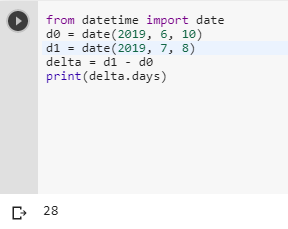2 views
in Python

If I have two dates (ex. '8/18/2008' and '9/26/2008'), what is the best way to get the number of days between these two dates?

by (106k points)

There are multiple ways of solving the above problem but I am discussing the best approach here:-

First thing you can do is take two date objects once you have two date objects, you can just subtract them and you will get the number of days between them below is the code how we will do this:-

from datetime import date

d0 = date(2019, 6, 10)

d1 = date(2019, 7, 8)

delta = d1 - d0

print(delta.days)